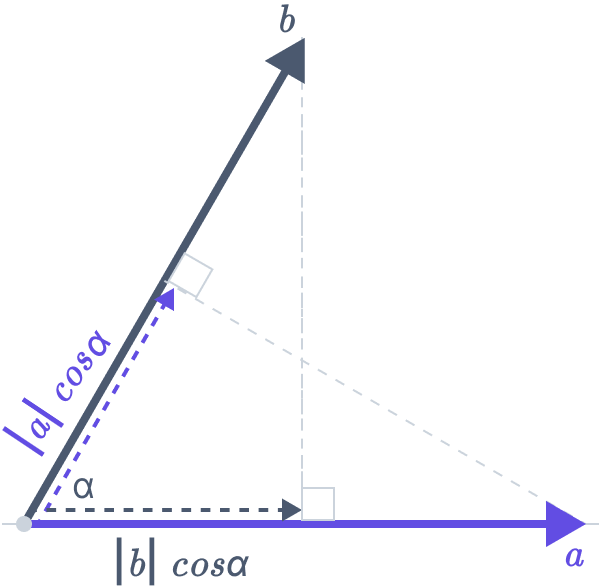# Dot Product Calculator

Dot Product Calculator shows the dot product of the specified vectors. The Mathematics Master online dot product calculator tool quickly displays the dot products and speeds up the calculation.Vector a

Vector b

## What is the Dot Product?

In mathematics, the scalar product is called the dot product. The scalar quantity that results from specified operations on the vector components is known as the dot product. The heavy dot is used to symbolize the dot product of two vectors.

## Dot Product Formula

The formula to determine the dot product of two vectors, if a and b are the two vectors, is given by

$$a·b = |a|·|b|·cos(θ)$$

Where:

• |a| and |b| are the magnitude of the vectors
• θ is the angle between the vectors

When cosine is the proportion of the scalar product to the magnitudes of the vectors, the calculator can also be used to determine the angle between two vectors:

$$cos(θ) = \dfrac{a × b}{|a| × |b|}$$

## Vector Dot Product Calculator

The two main types of vector multiplication are the cross product denoted by the symbol “×” and the dot product, commonly known as the scalar product represented by the symbol “,”. The crucial distinction is that the cross operation produces a vector, whereas the dot operation produces a single number as its product.

## How to Calculate the Dot Product of Two Vectors

The sum of the products in the cartesian coordinates of two vectors is known as a dot product. A dot product, often known as ab, is a single number rather than a vector, in contrast to the cross product.

If their dot product is zero, two vectors are orthogonal or perpendicular to one another.

## Example

Example 1: Calculate the dot product of: a = (2, 3, 4) and b = (5, −6, 7)

We need to use the component formula for the dot product of three-dimensional vectors here,

$$a⋅b = a_1 b_1 + a_2 b_2 + a_3 b_3$$

The dot product is:

$$a⋅b = 2(5) + 3(−6) + 4(7) = 10 − 18 + 28 = 20$$

Dot product = 20

## FAQs

How are matrices multiplied?

Matrix multiplication is not as straightforward as a scalar. The dot product creates the third matrix by joining two matrices—which need not be of the same dimension—into one. Alternatively, we can use a matrix dot product calculator.

What is the dot product of matrices?

Dot products are formed when the rows of the first matrix and the columns of the second matrix are multiplied. For example, the first step is the dot product between the first row of A and the first column of B. The member of the resulting matrix at position [0, 0] is the dot product’s output (i.e. first row, first column).